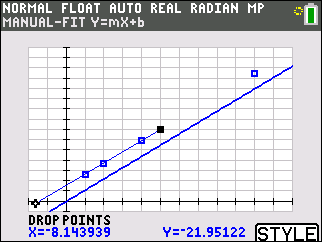Dinner Party

In this activity, students investigate the total cost of a private party at each of three restaurants. They will model the cost of a party at each restaurant with the graph of a linear function.
•TI-84 Plus CE
•TI-84 Plus C Silver Edition
• TI-84 Plus Silver Edition
• TI-84 PlusYou Are What You Eat

Students are introduced to modeling linear data through an investigation of comparing grams of fat and calories in fast food hamburgers.
•TI-84 Plus CE
•TI-84 Plus C Silver Edition
• TI-84 Plus Silver Edition
• TI-84 PlusPoints, Lines and Slopes (Oh My!)

In this activity students will explore the relationship between coordinates of points and locations on the coordinate plane, the relationships of lines with their equations, slopes and y-intercepts, and lastly, the slopes of parallel and perpendicular lines.
•TI-84 Plus CE
•TI-84 Plus C Silver Edition
• TI-84 Plus Silver Edition
• TI-84 Plus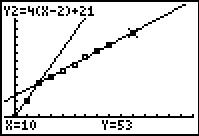Dog Days or Dog Years?

Students will use order pairs, table of values, and a scatter plot to determine a function that represents real world data.
•TI-84 Plus CE
• TI-84 PlusGuess My Coefficients

Students will represent and analyze mathematical situations and structures using algebraic symbols and understand patterns, relations, and functions.
• TI-84 Plus Silver Edition
• TI-84 Plus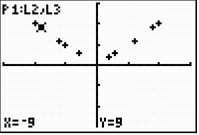Introducing the Absolute Value Function

Students will examine data by comparing individual data points to the mean by finding the difference (positive or negative) and the distance from the mean.

•TI-84 Plus CE
•TI-84 Plus C Silver Edition
• TI-84 Plus Silver Edition
• TI-84 Plus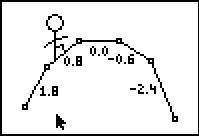Math Man On The Slopes

Students practice identifying slopes with informal pictures, and can self-check their understanding with one of the measurement tools.
• TI-84 Plus Silver Edition
• TI-84 Plus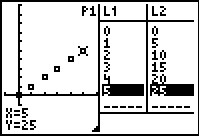Trains in Motion

Students will make observations about the motion of two objects. They will compare and contrast this motion and consider how it corresponds to a graph representing distance as a function of time.
•TI-84 Plus CE
•TI-84 Plus C Silver Edition
• TI-84 Plus Silver Edition
• TI-84 Plus